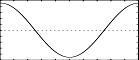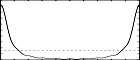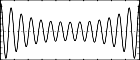## GEN11

GEN11 — Generates an additive set of cosine partials.

## Description

This subroutine generates an additive set of cosine partials, in the manner of Csound generators buzz and gbuzz.

## Syntax

`f # time size 11 nh [lh] [r]`

## Initialization

size -- number of points in the table. Must be a power of 2 or power-of-2 plus 1 (see f statement).

nh -- number of harmonics requested. Must be positive.

lh(optional) -- lowest harmonic partial present. Can be positive, zero or negative. The set of partials can begin at any partial number and proceeds upwards; if lh is negative, all partials below zero will reflect in zero to produce positive partials without phase change (since cosine is an even function), and will add constructively to any positive partials in the set. The default value is 1

r(optional) -- multiplier in an amplitude coefficient series. This is a power series: if the lhth partial has a strength coefficient of A the (lh + n)th partial will have a coefficient of A * rn, i.e. strength values trace an exponential curve. r may be positive, zero or negative, and is not restricted to integers. The default value is 1.

NoteThis subroutine is a non-time-varying version of the CSound buzzand gbuzz generators, and is similarly useful as a complex sound source in subtractive synthesis. With lh and r present it parallels gbuzz; with both absent or equal to 1 it reduces to the simpler buzz (i.e. nh equal-strength harmonic partials beginning with the fundamental). Sampling the stored waveform with an oscillator is more efficient than using the dynamic buzz units. However, the spectral content is invariant and care is necessary, lest the higher partials exceed the Nyquist during sampling to produce fold-over.

## Examples

Here is an example of the GEN11 routine. It uses the files gen11.csd.

Example 1277. An example of the GEN11 routine.

```<CsoundSynthesizer>
<CsOptions>
; Select audio/midi flags here according to platform
-odac      ;;;realtime audio out
; For Non-realtime ouput leave only the line below:
; -o gen11.wav -W ;;; for file output any platform
</CsOptions>
<CsInstruments>

sr = 44100
ksmps = 32
nchnls = 2
0dbfs  = 1

instr 1

ifn  = p4
asig oscil .8, 220, ifn
outs asig,asig

endin
</CsInstruments>
<CsScore>
f 1 0 16384 11 1 1	;number of harmonics = 1
f 2 0 16384 11 10 1 .7	;number of harmonics = 10
f 3 0 16384 11 10 5 2	;number of harmonics = 10, 5th harmonic is amplified 2 times

i 1 0 2 1
i 1 + 2 2
i 1 + 2 3
e
</CsScore>
</CsoundSynthesizer>
```

These are the diagrams of the waveforms of the GEN11 routines, as used in the example:f 1 0 16384 11 1 1f 2 0 16384 11 10 1 .7f 3 0 16384 11 10 5 2